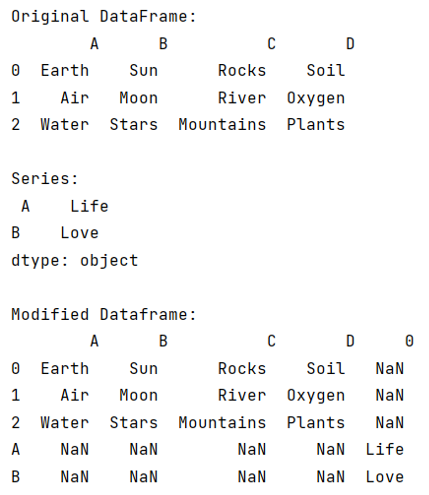# How to Merge a Series and DataFrame?

Given a Series and DataFrame, we have to merge them.
Submitted by Pranit Sharma, on July 04, 2022

Both DataFrame and series are the two main data structures of the pandas library. A Series in pandas contains a single list that can store heterogeneous types of data, because of this, a series is also considered as a 1-dimensional data structure.

On the other hand, DataFrame is a 2-dimensional data structure that contains multiple lists of the heterogeneous type of data. DataFrame can contain multiple series or it can be considered as a collection of series.

When we analyze a series, each value can be considered as a separate row of a single column. In contrast, when we analyze a DataFrame, we have multiple columns and multiple rows.

Both series and DataFrame can be created either with the list or with the help of a dictionary, in the case of series, there will be only one key in the dictionary but there may be multiple keys in the case of DataFrame.

To merge Series and DataFrame, we will first convert Series into DataFrame by using series.to_frame() method and then we will merge the two frames by using pandas.concat() method.

To work with pandas, we need to import pandas package first, below is the syntax:

```import pandas as pd
```

Let us understand with the help of an example,

## Python code to merge a series and dataframe

```# Importing pandas package
import pandas as pd

# Creating a dictionary
d = {
'A':['Earth','Air','Water'],
'B':['Sun','Moon','Stars'],
'C':['Rocks','River','Mountains'],
'D':['Soil','Oxygen','Plants']
}

# Creating a DataFrame
df = pd.DataFrame(d)

# Creating a Series
s = pd.Series({'A':'Life','B':'Love'})

# Display original DataFrame
print("Original DataFrame:\n",df,"\n")

# Display Series
print("Series:\n",s,"\n")

# Converting series into DataFrame
s_f = s.to_frame()

# Encapsulating both frames in a list
frames = [df,s_f]

# concatenating series and dataframe
df = pd.concat(frames)

# Display result
print("Modified Dataframe:\n",df)
```

Output:Languages: » C » C++ » C++ STL » Java » Data Structure » C#.Net » Android » Kotlin » SQL
Web Technologies: » PHP » Python » JavaScript » CSS » Ajax » Node.js » Web programming/HTML
Solved programs: » C » C++ » DS » Java » C#
Aptitude que. & ans.: » C » C++ » Java » DBMS
Interview que. & ans.: » C » Embedded C » Java » SEO » HR
CS Subjects: » CS Basics » O.S. » Networks » DBMS » Embedded Systems » Cloud Computing
» Machine learning » CS Organizations » Linux » DOS
More: » Articles » Puzzles » News/Updates